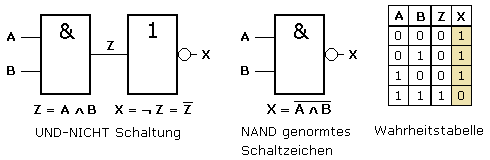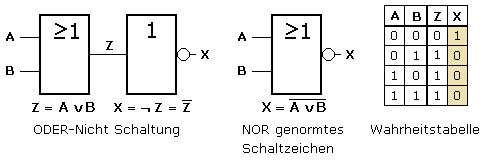# What does that mean in logic circuits

### NAND operation - negated AND

A combination of an AND gate followed by a NOT gate results in a NAND gate. The output variable Z of the AND gate is negated by the NOT gate and generates the output variable X of the NAND gate. Many logical functions can be solved by using NAND gates. This often increases the number of gates required, with the economic advantage of only using one type of gate.The output state of a NAND gate results in 1 if not all input states are 1.

### NOR link - negated OR

A combination of an OR gate followed by a NOT gate results in a NOR gate. The output variable Z of the OR gate is negated by the NOT gate and generates the output variable X of the NOR gate. NOR gates have the same important meaning as NAND gates in logic circuits.The output state of a NOR element results in 1 if all input states are 0.

### XOR link - Exclusive OR - Antivalence

The logical connection of the XOR gate with two input variables can be described with an 'either - or'. The output variable will always return 'true' if the input variables have different states. The truth table of the XOR gate corresponds to the OR gate with the exclusion of identical input states, i.e. excluding equivalence. This behavior is known as antivalence. There is a circuit symbol standardized in accordance with IEC 60617-12.

If the v is used as a character in the functional equation for the OR link, the XOR link can be underlined by an v be marked. When using the + sign for OR, a plus in the circle ⊕ indicates the XOR link.The function can be achieved by a circuit with four NAND gates. The following video clip shows the signal flow for the four possible input states for a circuit simulation. Individual control is possible with the help of the fade-in control bar. Circuits with the combination of several NOT, AND and OR gates are uneconomical and are not used in logic circuits.

The output state of an XOR element results in 1 if an odd number of the input states is 1
and all other input states are 0.